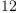login  home  contents  what's new  discussion  bug reports help  links  subscribe  changes  refresh  edit

f calculates the number of 0-1 matrices with row sums A and column sums B.

fricas
f(A:List PI, B:List PI): FRAC INT == cap(reduce(*, [elementary i for i in A]), reduce(*, [complete i for i in B]))
Function declaration f : (List(PositiveInteger), List(
PositiveInteger)) -> Fraction(Integer) has been added to
workspace.
Type: Void

For example, there are

fricas
f([2,2,2,2], [2,3,3])
fricas
Compiling function f with type (List(PositiveInteger), List(
PositiveInteger)) -> Fraction(Integer)(1)
Type: Fraction(Integer)

0-1 matrices whose rows all sum up to two and whose first column has 2 entries equal to one, the others having 3 entries equal to one.

 Subject:   Be Bold !! ( 14 subscribers )